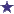# Ratio/Proportion Question about Amox/Clav 600mg/5ml to 400mg/5ml

by Veronica
(Albany, NY)Mechanics of Action of an Antibiotics

I have a ratio/proportion question that calls for Amoxicillin/Clavulanate 600mg/5ml for 4ml PO twice daily. However, we are out of stock of the 600mg/5ml concentration. On hand I have 400mg/5ml how can I solve this problem.

Average RatingRatingby: David Let's figure out the strength of each dose first. 600mg/5ml = 120mg/1ml 120mg/1ml X 4ml = 480mg is the total twice daily dose. Veronica has the 400mg/5ml in stock. Now, we need to figure out how much of the reconstituted liquid that we will need to give to equal 480mg. 400mg/5ml = 80mg/1ml 480mg divided by 80mg/1ml = 6ml 6ml X 80mg/1ml = 480mg/6ml